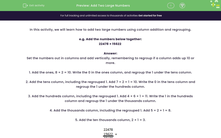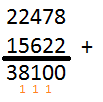# Add Two Large Numbers Using the Column Method and Exchanging

In this worksheet, students will use the column method to add together large numbers resulting in 5-digit and 6-digit answers.Key stage:  KS 2

Curriculum topic:   Number: Addition and Subtraction

Curriculum subtopic:   Add/Subtract Four-Digit (or more) Numbers

Popular topics:   Subtraction worksheets, Addition worksheets

Difficulty level:#### Worksheet Overview

In this activity, we will learn how to add two large numbers using column addition and regrouping (also known as carrying).Example

22,478 + 15,622

Set the numbers out in columns and add vertically, remembering to regroup (or carry) if a column adds up to 10 or more.

1. Add the ones: 8 + 2 = 10. Write the 0 in the ones column, and regroup (or carry) the 1 under the tens column.

2. Add the tens column, including the regrouped 1. Add 7 + 2 + 1 = 10. Write the 0 in the tens column and regroup the 1 under the hundreds column.

3. Add the hundreds column, including the regrouped 1. Add 4 + 6 + 1 = 11. Write the 1 in the hundreds column and regroup the 1 under the thousands column.

4. Add the thousands column, including the regrouped 1. Add 5 + 2 + 1 = 8.

5. Add the ten thousands column: 2 + 1 = 3.In summary:

22,478 + 15,622 = 38,100

Are you happy to have a go at some questions now?### What is EdPlace?

We're your National Curriculum aligned online education content provider helping each child succeed in English, maths and science from year 1 to GCSE. With an EdPlace account you’ll be able to track and measure progress, helping each child achieve their best. We build confidence and attainment by personalising each child’s learning at a level that suits them.

Get started